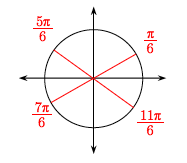### Home > INT3 > Chapter Ch12 > Lesson 12.1.4 > Problem12-59

12-59.

Solve each equation below for $x$ if $0\le x<2\pi$. Note that $\sin^2(x)$ is the notation that is used for $(\sin(x))^2$.

1. $4\sin^2(x)=1$

Divide both sides by $4$.

$\sin^2(x)=\frac{1}{4}$

Take the square root of both sides to solve for $\sin(x)$.

$\sin(x)=±\frac{1}{2}$

Find the corresponding angles on the unit circle.1. $3\tan^2(x)=1$

Follow the steps in part (a).